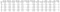# Introduction

As the quarantine continues, I’ve picked up a number of hobbies and interests… including WINE. Recently, I’ve acquired a taste for wines, although I don’t really know what makes a good wine. Therefore, I decided to apply some machine learning models to figure out what makes a good quality wine!

For this project, I used Kaggle’s Red Wine Quality dataset to build various classification models to predict whether a particular red wine is “good quality” or not. Each wine in this dataset is given a “quality” score between 0 and 10. For the purpose of this project, I converted the output to a binary output where each wine is either “good quality” (a score of 7 or higher) or not (a score below 7). The quality of a wine is determined by 11 input variables:

1. Fixed acidity
2. Volatile acidity
3. Citric acid
4. Residual sugar
5. Chlorides
6. Free sulfur dioxide
7. Total sulfur dioxide
8. Density
9. pH
10. Sulfates
11. Alcohol

## Objectives

The objectives of this project are as follows

1. To experiment with different classification methods to see which yields the highest accuracy
2. To determine which features are the most indicative of a good quality wine

With that said, here we go!

# Setup

First, I imported all of the relevant libraries that I’ll be using as well as the data itself.

## Importing Libraries

import numpy as np
import pandas as pd
import matplotlib as plt
import seaborn as sns
import plotly.express as px

## Understanding Data

Next, I wanted to get a better idea of what I was working with.

# See the number of rows and columns

print("Rows, columns: " + str(df.shape))# See the first five rows of the dataset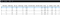There are a total of 1599 rows and 12 columns. The data looks very clean by looking at the first five rows, but I still wanted to make sure that there were no missing values.

# Missing Values

print(df.isna().sum())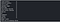This is a very beginner-friendly dataset. I did not have to deal with any missing values, and there isn’t much flexibility to conduct some feature engineering given these variables. Next, I wanted to explore my data a little bit more.

# Exploring Variables

## Histogram of ‘quality’ variable

First, I wanted to see the distribution of the quality variable. I wanted to make sure that I had enough ‘good quality’ wines in my dataset — you’ll see later how I defined ‘good quality’.

fig = px.histogram(df,x=‘quality’)
fig.show()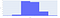## Correlation Matrix

Next I wanted to see the correlations between the variables that I’m working with. This allows me to get a much better understanding of the relationships between my variables in a quick glimpse.

Immediately, I can see that there are some variables that are strongly correlated to quality. It’s likely that these variables are also the most important features in our machine learning model, but we’ll take a look at that later. Go through our ML Course to learn ML algorithms, statistics, supervised and unsupervised learning, etc.

corr = df.corr()
matplotlib.pyplot.subplots(figsize=(15,10))
sns.heatmap(corr, xticklabels=corr.columns, yticklabels=corr.columns, annot=True, cmap=sns.diverging_palette(220, 20, as_cmap=True))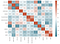# Convert to a Classification Problem

Going back to my objective, I wanted to compare the effectiveness of different classification techniques, so I needed to change the output variable to a binary output.

For this problem, I defined a bottle of wine as ‘good quality’ if it had a quality score of 7 or higher, and if it had a score of less than 7, it was deemed ‘bad quality’.

Once I converted the output variable to a binary output, I separated my feature variables (X) and the target variable (y) into separate dataframes.

# Create Classification version of target variable

df[‘goodquality’] = [1 if x >= 7 else 0 for x in df[‘quality’]]# Separate feature variables and target variable
X = df.drop([‘quality’,‘goodquality’], axis = 1)
y = df[‘goodquality’]

## Proportion of Good vs Bad Wines

I wanted to make sure that there was a reasonable number of good quality wines. Based on the results below, it seemed like a fair enough number. In some applications, resampling may be required if the data was extremely imbalanced, but I assumed that it was okay for this purpose.

# See proportion of good vs bad wines

df[‘goodquality’].value_counts()# Preparing Data for Modelling

## Standardizing Feature Variables

At this point, I felt that I was ready to prepare the data for modelling. The first thing that I did was standardize the data. Standardizing the data means that it will transform the data so that its distribution will have a mean of 0 and a standard deviation of 1. It’s important to standardize your data in order to equalize the range of the data.

For example, imagine a dataset with two input features: height in millimeters and weight in pounds. Because the values of ‘height’ are much higher due to its measurement, a greater emphasis will automatically be placed on height than weight, creating a bias.

# Normalize feature variables

from sklearn.preprocessing import StandardScaler
X_features = X
X = StandardScaler().fit_transform(X)

## Split data

Next I split the data into a training and test set so that I could cross-validate my models and determine their effectiveness.

# Splitting the data

from sklearn.model_selection import train_test_split
X_train, X_test, y_train, y_test = train_test_split(X, y, test_size=.25, random_state=0)

Now, comes the fun part!

# Modelling

For this project, I wanted to compare five different machine learning models: decision trees, random forests, AdaBoost, Gradient Boost, and XGBoost. For the purpose of this project, I wanted to compare these models by their accuracy.

## Model 1: Decision Tree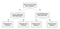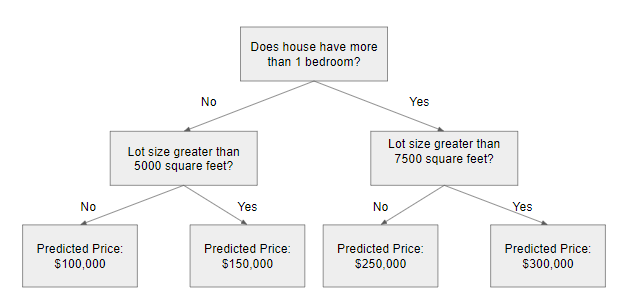Image created by author

Decision trees are a popular model, used in operations research, strategic planning, and machine learning. Each square above is called a node, and the more nodes you have, the more accurate your decision tree will be (generally). The last nodes of the decision tree, where a decision is made, are called the leaves of the tree. Decision trees are intuitive and easy to build but fall short when it comes to accuracy.

from sklearn.metrics import classification_report
from sklearn.tree import DecisionTreeClassifiermodel1 = DecisionTreeClassifier(random_state=1)
model1.fit(X_train, y_train)
y_pred1 = model1.predict(X_test)print(classification_report(y_test, y_pred1))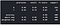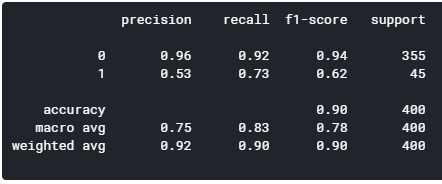## Model 2: Random Forest

Random forests are an ensemble learning technique that builds off of decision trees. Random forests involve creating multiple decision trees using bootstrapped datasets of the original data and randomly selecting a subset of variables at each step of the decision tree. The model then selects the mode of all of the predictions of each decision tree. What’s the point of this? By relying on a “majority wins” model, it reduces the risk of error from an individual tree.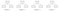Image created by author

For example, if we created one decision tree, the third one, it would predict 0. But if we relied on the mode of all 4 decision trees, the predicted value would be 1. This is the power of random forests.

from sklearn.ensemble import RandomForestClassifier
model2 = RandomForestClassifier(random_state=1)
model2.fit(X_train, y_train)
y_pred2 = model2.predict(X_test)print(classification_report(y_test, y_pred2))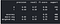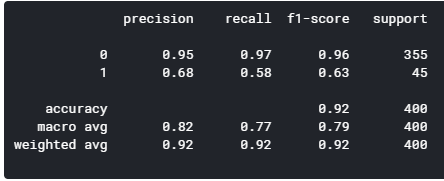The next three models are boosting algorithms that take weak learners and turn them into strong ones. I don’t want to get sidetracked and explain the differences between the three because it’s quite complicated and intricate. That being said, I’ll leave some resources where you can learn about AdaBoost, Gradient Boosting, and XGBoosting.

model3.fit(X_train, y_train)
y_pred3 = model3.predict(X_test)print(classification_report(y_test, y_pred3))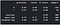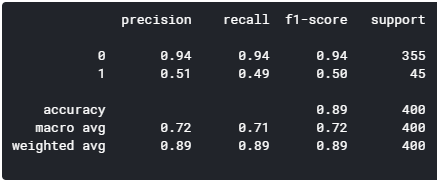model4.fit(X_train, y_train)
y_pred4 = model4.predict(X_test)print(classification_report(y_test, y_pred4))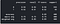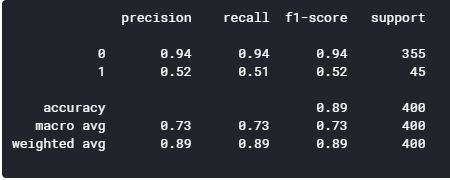## Model 5: XGBoost

import xgboost as xgb
model5 = xgb.XGBClassifier(random_state=1)
model5.fit(X_train, y_train)
y_pred5 = model5.predict(X_test)print(classification_report(y_test, y_pred5))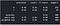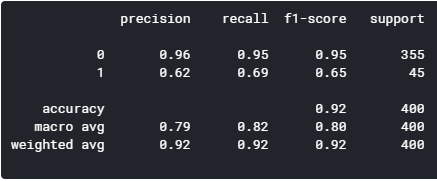By comparing the five models, the random forest and XGBoost seems to yield the highest level of accuracy. However, since XGBoost has a better f1-score for predicting good quality wines (1), I’m concluding that the XGBoost is the winner of the five models.

# Feature Importance

Below, I graphed the feature importance based on the Random Forest model and the XGBoost model. While they slightly vary, the top 3 features are the same: alcohol, volatile acidity, and sulphates. If you look below the graphs, I split the dataset into good quality and bad quality to compare these variables in more detail.

## via Random Forest

feat_importances = pd.Series(model2.feature_importances_, index=X_features.columns)
feat_importances.nlargest(25).plot(kind=‘barh’,figsize=(10,10))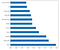## via XGBoost

feat_importances = pd.Series(model5.feature_importances_, index=X_features.columns)
feat_importances.nlargest(25).plot(kind=‘barh’,figsize=(10,10))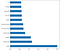# Filtering df for only good quality

df_temp = df[df[‘goodquality’]==1]
df_temp.describe()# Filtering df for only bad quality
df_temp2 = df[df[‘goodquality’]==0]
df_temp2.describe()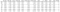Good quality